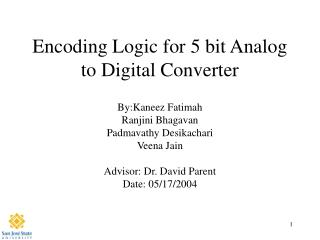DownloadDownload PresentationEncoding Logic for 5 bit Analog to Digital Converter

# Encoding Logic for 5 bit Analog to Digital Converter

Download Presentation## Encoding Logic for 5 bit Analog to Digital Converter

- - - - - - - - - - - - - - - - - - - - - - - - - - - E N D - - - - - - - - - - - - - - - - - - - - - - - - - - -
##### Presentation Transcript

1. Encoding Logic for 5 bit Analog to Digital Converter By:Kaneez Fatimah Ranjini Bhagavan Padmavathy Desikachari Veena Jain Advisor: Dr. David Parent Date: 05/17/2004

2. Agenda • Abstract • Introduction - Why this Project • ADC Encoding Logic • Project Details • Results • Cost Analysis • Summary

3. Abstract • Designed Encoding logic for 5 bit Analog to Digital Converter without Sample and Hold operation • Used AMI06 process • Clock frequency = 200 MHz • Power ~ 3mW@200MHz

4. Why this Project • This project is based on IEEE Research paper on enhanced ADC logic without sample & hold • Needed interpolation from basic 8 bit circuit to 5 bit logic • Dr. Parent suggested to implement this idea 

5. Encoding Logic • The design of converter is optimized to minimize the effect of errors that may occur in ADC architecture without a sample & hold • Two levels of Encoding • Total Comparators # 32 • First Encoding Level for n = 1 to 8 is given by B[n] = {C[n] and (Not C[n+8])} OR {C[n+16] and (Not C[n+24])} B = {C and (Not C)}OR {C and (Not C)} Comparators First Encoding Level Second Encoding Level CM1 B1 Bit0 Bit1 CM32 B9 Bit4

6. Encoding Logic Contd.. • Second Level of Encoding – • Bit 0 = (B1 XOR B2) OR (B3 XOR B4) OR (B5 XOR B6) OR (B7 XOR B8) • Bit 1 = (B2 XOR B4) OR (B6 XOR B8) • Bit 2 = (B4 XOR B8) • Bit 3 = B8 • Bit4 = B9

7. Project Details • Comparator output Simulated • First Level Encoding - Inverter, AOI22 • Second Level Encoding - 2 input XOR, 2input OR , 4input OR • Flipflop

8. Schematic

9. Verilog Simulation

10. Longest Path Calculations

11. Transient Analysis

12. Propagation Delay

13. Layout

14. DRC Check & LVS

15. Cost Analysis • Time we spent on each phase of the project • verifying logic : 6 Hours • verifying timing : 20 Hours • layout : 30 Hours • post extraction : 1 Hour

16. Summary • Designed Encoding Logic for 5 bit Analog to Digital Converter without Sample & Hold. • Designed Conforming to Specifications • Clock frequency > 200 MHz • Power < 3mW • Area : 337.2 um x 104.70 um

17. Reference • IEEE Paper : An 8-bit 250 megasample per second analog-to-digital converter: operation without a sample and holdPeetz, B.   Hamilton, B.D.   Kang, J.   This paper appears in: Solid-State Circuits, IEEE Journal of

18. Acknowledgements • Thanks to Dr. David Parent • Thanks to Cadence Design Systems for the VLSI lab